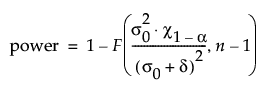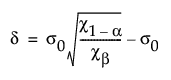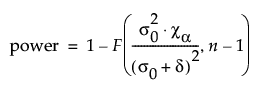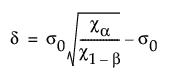Design of Experiments Guide > Prospective Sample Size and Power > One Sample Standard Deviation Calculator > Statistical Details for the One Sample Standard Deviation Calculator
Publication date: 11/10/2021

## Statistical Details for the One Sample Standard Deviation Calculator

The formula that JMP uses to calculate power depends on the setting of the Alternative Standard Deviation option.

For cases where the alternative standard deviation is larger, JMP calculates power as follows:where:

σ0 is the hypothesized standard deviation.

σ is the true standard deviation

Χp is the (1 - p)th quantile of the Χn-12 distribution.

F(x, n-1) is the cumulative distribution function of the Χn-12 distribution evaluated at x.

For cases where the alternative standard deviation is smaller, JMP calculates power as follows:where:

σ0 is the hypothesized standard deviation.

σ is the true standard deviation

Χp is the (1 - p)th quantile of the Χn-12 distribution.

F(x, n-1) is the cumulative distribution function of the Χn-12 distribution evaluated at x.

Numerical solutions are used to solve for n.